# Substitution

Our substitution feature allows you to answer the question "What would be the value of this expression if we substituted a different value for A?," without ever changing the original value of A.

Using the ‘with’ substitution function, you can replace a variable in an expression with a constant value, another variable expression, or a list.

You can find this function in the “Advanced” section of the “Functions” menu on the keypad, or ‘with’ can also be typed directly into an expression line using your keyboard.

### Substitution within a Function

Where would the parabola $$f\left(x\right)=ax^{2}+bx+c$$ move if $$b$$ were $$-1$$? Model $$f\left(x\right)$$ after substituting $$b$$ with $$-1$$ to visualize the change.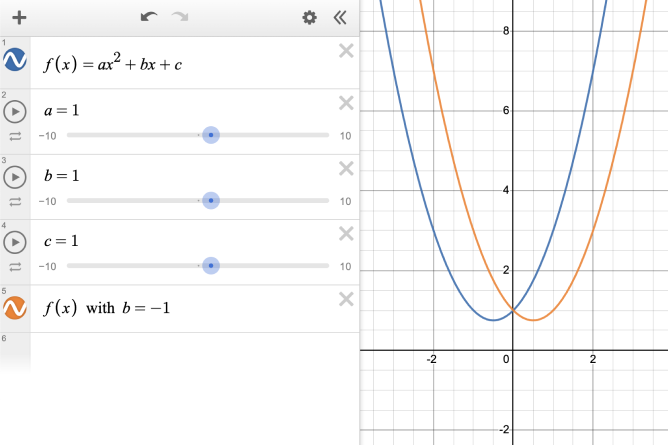Next, trying graphing the vertex of the original function $$(V)$$ and using substitution to also graph the vertex when $$b = -1$$.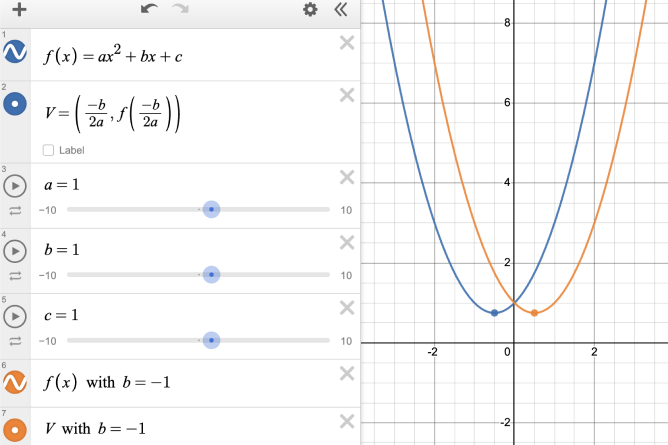### Substitution with a List

How do changes to $$a$$, $$b$$, and $$c$$ affect the path of the vertex of the parabola? By substituting $$b$$ with a list, you can model the path that the vertex would take.

First, graph the vertex of the original function $$(V)$$. Then, try substituting $$b$$ with a list of possible values.

You try! Open the graph below and try it out. Use the sliders to change the values of $$a$$, $$b$$, and $$c$$ and see how they compare to the path graphed in line 6. Example Graph.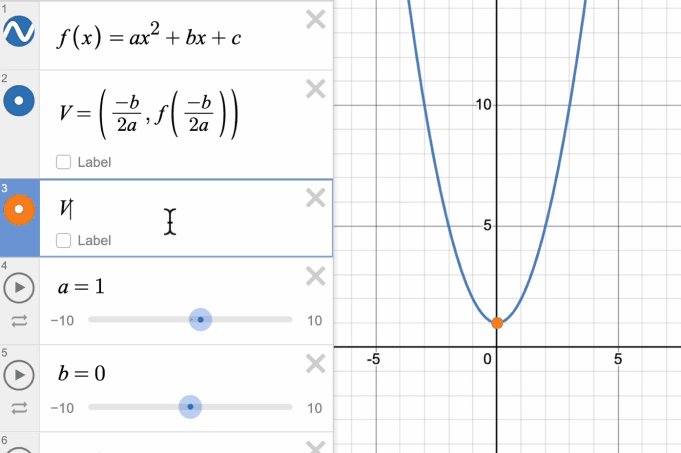### Substitution within the Same Expression Line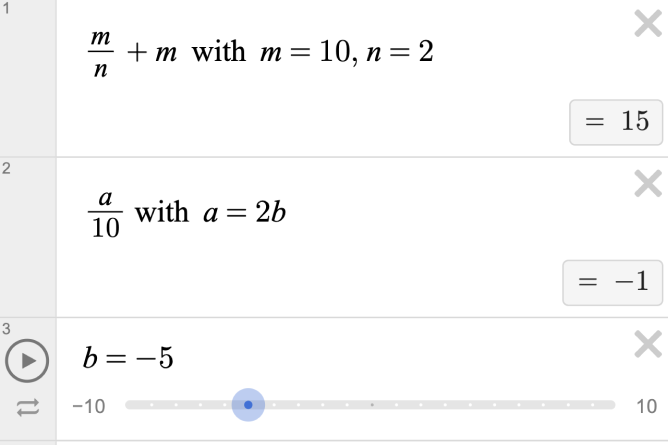You can evaluate expressions by substituting variables with constant values in the same line. You can also substitute a variable with another expression, but if that expression depends on a free variable, it will need to be defined separately.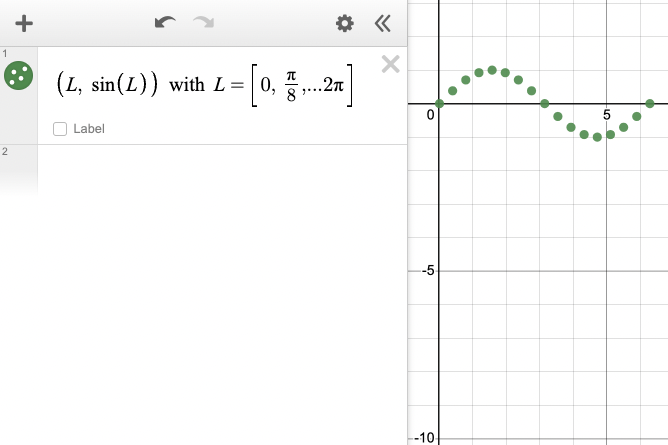By substituting the variable $$L$$ with a list of values, you can quickly plot points along the function $$y=sin(x)$$